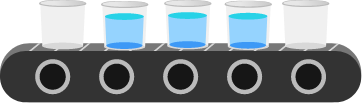### Computer Memory

This quiz is a quick overview and refresher of the concepts from discrete math needed to understand memory. In particular, the number bases 2 and 16, and converting into and out of various bases are important concepts for working with memory.

If we have a device that can be in one of two states, we can store a single digit of a base 2, or binary, number on that device. If we have many of these devices, we can store larger numbers, one binary digit per device.

In the picture below, we have a machine with buckets on a conveyor belt. To store a number, the machine fills certain buckets with water. We can consider this machine as representing a base 2 number, where each bucket represents a digit. Filled buckets represent a 1 and empty buckets represent a 0.What number is stored on the machine? Answer in base 10.

Whether it’s stored in RAM, in a bank of vacuum tubes, or on a conveyor belt of water buckets, digital data in its most basic form is just a series of individual binary digits, also known as bits. One bit represents one digit in base 2, which is either 0 or 1.

In this course, we’ll adopt the convention used in some programming languages to prefix binary numbers with 0b. What is the base 10 number 173 in binary? Prefix the binary with 0b.

How many bits are required to represent 128 in binary?

We can determine the largest base 10 value that can be represented by a certain number of bits in binary by setting all of the bits to 1 and converting to base 10. What’s the largest base 10 number we can represent with 8 bits?

8 bits are referred to as a byte. As we saw in the last problem, the largest number that can be stored in a single byte is 255. Clearly, we require three digits to represent 255 in base 10. What is the smallest base that can represent 255 using only 2 digits?

Base 16 is also referred to as hexadecimal, or hex for short. In this course, we’ll adopt the convention used in some programming languages to prefix hexadecimal numbers with 0x. For example, since 32 in decimal is $2 \times 16^1 + 0 \times 16^0$, it will be represented as 0x20.

Since one hexadecimal digit needs to represent up to the value 15 in decimal, we will use letters for some of the digits:

$\begin{array} { | c | c| } \hline \text{dec} & \text{hex} \\ \hline 0 & 0 \\ 1 & 1 \\ 2 & 2 \\ \vdots & \vdots \\ 9 & 9 \\ 10 & \text{A} \\ 11 & \text{B} \\ 12 & \text{C} \\ 13 & \text{D} \\ 14 & \text{E} \\ 15 & \text{F} \\ \hline \end{array}$

$\begin{array} { | c c r c | } \hline \text{dec} & & & & \text{hex} \\ \hline 0 &= & 0 \times 16^0 & = & 0\text{x}0 \\ 1 &= & 1 \times 16^0 & = & 0\text{x}1 \\ 9 &= & 9 \times 16^0 & = & 0\text{x}9 \\ 10 &= & 10 \times 16^0 & = & 0\text{xA} \\ 11 &= & 11 \times 16^0 & = & 0\text{xB} \\ 12 &= & 12 \times 16^0 & = & 0\text{xC} \\ 15 &= & 15 \times 16^0 & = & 0\text{xF} \\ 16 &= & 1\times 16^1 + 0 \times 16^0 & = & 0\text{x}10 \\ 17 &= & 1\times 16^1 + 1 \times 16^0 & = & 0\text{x}11 \\ 25 &= & 1\times 16^1 + 9 \times 16^0 & = & 0\text{x}19 \\ 26 &= & 1\times 16^1 + 10 \times 16^0 & = & 0\text{x}1\text{A} \\ 31 &= & 1\times 16^1 + 15 \times 16^0 & = & 0\text{x}1\text{F} \\ 32 &= & 2\times 16^1 + 0 \times 16^0 & = & 0\text{x}20 \\ 159 &= & 9\times 16^1 + 15 \times 16^0 & = & 0\text{x}9\text{F} \\ 160 &= & 10\times 16^1 + 0 \times 16^0 & = & 0\text{xA}0 & \\ 255 &= & 15\times 16^1 + 15 \times 16^0 & = & 0\text{xFF} \\ \hline \end{array}$

The decimal number 47872 is represented as 0xBB00 in hexadecimal. What is the decimal number 48000 in hexadecimal?

As we saw in the previous question, 255 in hexadecimal is 0xFF. This happens to be the maximum possible value that can be represented by 2 digits in hexadecimal. Recall that 255 in binary is 0b11111111, which is also the maximum possible value that can be represented by 8 digits in binary.

This property makes hexadecimal invaluable in computer science. It allows us to concatenate bytes in either base and get the same result. For example:

$\begin{array} { | c | c| c | } \hline \text{Binary} & \text{Hexadecimal} & \text{Decimal} \\ \hline \text{0b11101111} & \text{0xEF} & 239 \\ \text{0b00111001} & \text{0x39} & 057 \\ \hline \end{array}$

$\text{0b1110111100111001} = 61241$,
$\text{0xEF39} = 61241$.

Same result. But if we concatenate the two decimal values, we get something completely different: 239057.

We normally deal with integers of fixed sizes in multiples of bytes. Recall that a byte is 8 bits. It’s common to see 8-bit, 16-bit, 32-bit, and 64-bit integers in contemporary code. The largest value of a 32-bit integer is $2^{32} - 1$ which is 4294967295 in decimal. If we store a much smaller number, the remaining unused bits must be set to 0, since sizes are fixed.

What is the decimal value 763 stored in a 32-bit integer, padding the unused bits with 0s? Answer in hexadecimal.